Home

# THE COMPLEXITY OF THOMASON’S ALGORITHM FOR FINDING A SECOND HAMILTONIAN CYCLE

## Abstract

By Smith’s theorem, if a cubic graph has a Hamiltonian cycle, then it has a second Hamiltonian cycle. Thomason [‘Hamilton cycles and uniquely edge-colourable graphs’, Ann. Discrete Math. 3 (1978), 259–268] gave a simple algorithm to find the second cycle. Thomassen [private communication] observed that if there exists a polynomially bounded algorithm for finding a second Hamiltonian cycle in a cubic cyclically 4-edge connected graph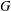$G$ , then there exists a polynomially bounded algorithm for finding a second Hamiltonian cycle in any cubic graph$G$ . In this paper we present a class of cyclically 4-edge connected cubic bipartite graphs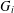$G_{i}$ with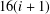$16(i+1)$ vertices such that Thomason’s algorithm takes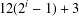$12(2^{i}-1)+3$ steps to find a second Hamiltonian cycle in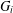$G_{i}$ .

## References

Hide All
MathJax
MathJax is a JavaScript display engine for mathematics. For more information see http://www.mathjax.org.

# THE COMPLEXITY OF THOMASON’S ALGORITHM FOR FINDING A SECOND HAMILTONIAN CYCLE

## Metrics

### Full text viewsFull text views reflects the number of PDF downloads, PDFs sent to Google Drive, Dropbox and Kindle and HTML full text views.

Total number of HTML views: 0
Total number of PDF views: 0 *Loading metrics...

### Abstract viewsAbstract views reflect the number of visits to the article landing page.

Total abstract views: 0 *Loading metrics...

* Views captured on Cambridge Core between <date>. This data will be updated every 24 hours.

Usage data cannot currently be displayed